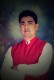# How can I get calculate result valur of multiple questions? My form id 91855461137158

•Dr sanjeev kumar
Asked on July 10, 2019 at 05:44 AM•AndrewHag
Answered on July 10, 2019 at 06:36 AM

It seems you have already calculated the results using the form calculation widget. And it seems you are trying to get the absolute value of the results using the function abs(). For that, you need to write the calculation formula within the function abs(write the formula here).

Let us know if you need any further assistance.